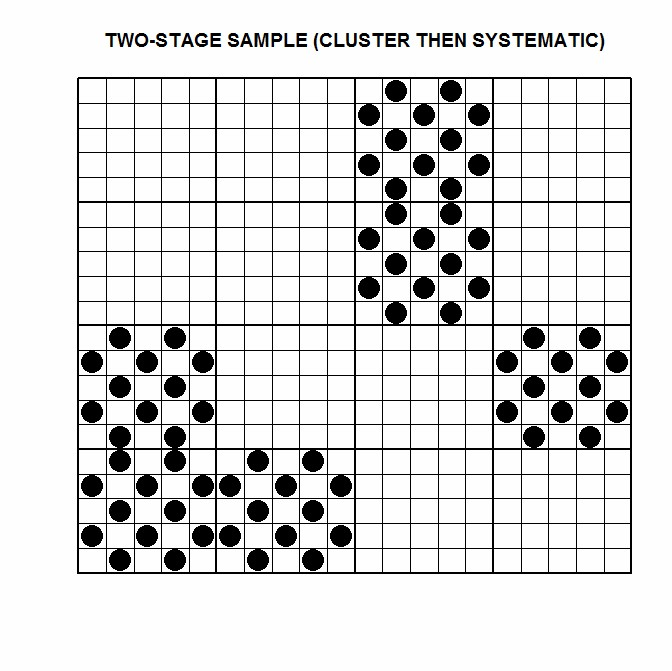# Survey Sampling Methods

Data Collection Techniques

The sampling method(s) used for a survey depends on several factors including:
• Budgetary constraints and available resources
• Information to be gathered from the survey
• Information known prior to running the survey

The following are the main survey sampling methods.

Simple Random Sample

This involves taking a random sample of n units from a population of size N.

In the following diagram, 48 units are randomly sampled from the survey population of 400.Disadvantage: It may not be as accurate as stratified sampling or as cheap as cluster sampling.

Stratified Sample

This involves dividing the population into non-overlapping blocks (strata) and taking a random sample(s) based around these blocks.  Stratified sampling can be done using either pre-stratification or post-stratification.

For pre-stratification, a random sample would be taken within each stratum.

For post-stratification, a random sample would be taken, and then estimates made from that sample would be weighted according to known population figures.

In the following diagram, 3 units are randomly sampled from each of the 16 groups.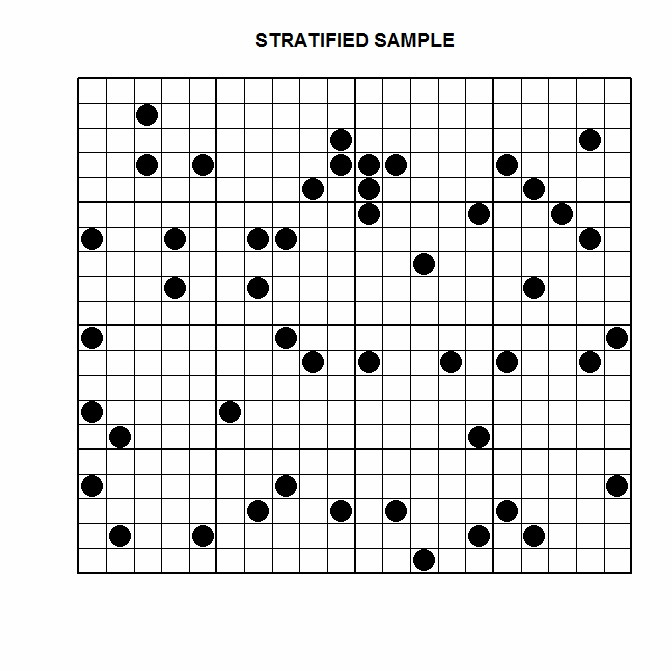Example 1:

It is known that 50% of a population are male, and 50% are female.  People from the population are surveyed, to estimate something e.g. their employment status, opinions, buying habits etc.

If pre-stratification is used, two random samples are made – one for males, and one for females.  The sizes of each sample would ideally be equal.

If post-stratification is used, a single random sample is made with the sex of the survey participant noted.  A weighted estimate is made, to ensure proportional representation.

Example 2:

The demographic breakdown of a population is known, which includes sex, race and age group.  People from the population are surveyed to estimate something.

If pre-stratification is used, a random sample might be made for every combination of characteristics e.g. Asian females in their 20s, Caucasian males in their 30s etc.  The size of each sample would ideally be proportional to their population size.

If post-stratification is used, a single random sample is made with the sex, race and age group of the survey participant noted.  A computational technique known as rim-weighting (or raking) might be used, to form weights.  The weights would then used to make weighted estimates.

Cluster Sample

This involves sampling every unit from several randomly selected clusters.  Like with stratified sampling, it involves dividing the population into non-overlapping blocks (clusters).  The clusters usually relate to geographic proximity.

In the following diagram, 3 of the 16 available clusters were randomly sampled.Disadvantage: If the variation between clusters is great relative to the variation within clusters, cluster sampling can result in inaccurate estimates.

Example 1:

A survey is to be done, using individual households from a city as the sampling units.  The participants would be surveyed in person by an interviewer.

Instead of taking a simple random sample of households, it would be cheaper and less time consuming, to take a random sample of streets from the city, and then sample every household from that street.

Example 2:

A survey is to be done, using individual students from a school as the sampling unit.

Instead of taking a large random sample of students from the school, it may be quicker (and cheaper) to sample every student from several randomly selected class rooms.

Systematic Sample

This involves sampling every kth unit from a listed population.

In the following diagram, every 10th unit is sampled.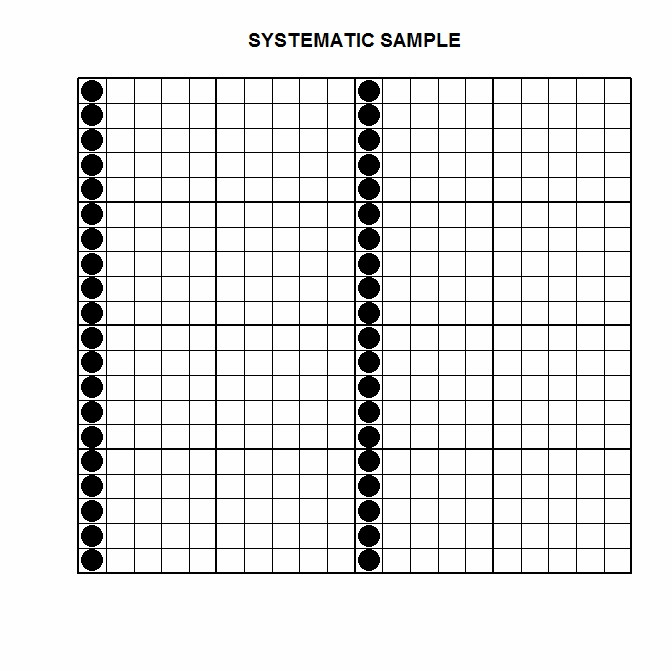In the following diagram, every 6th unit is sampled.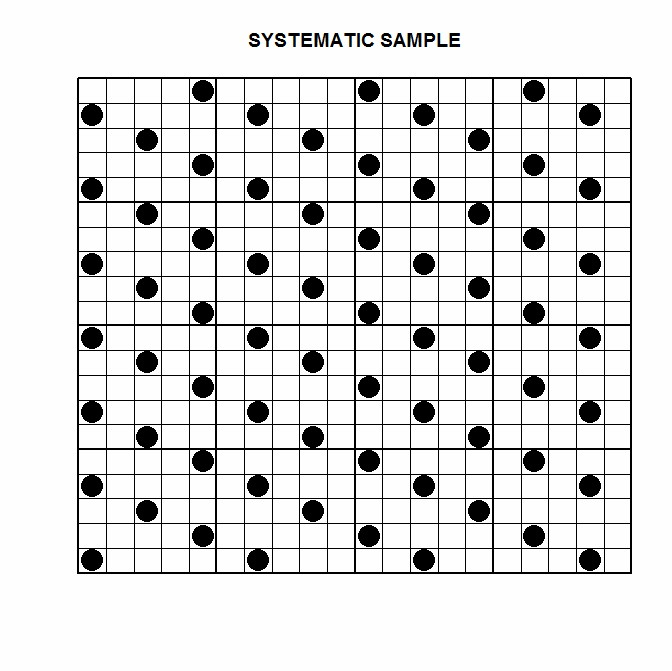Advantage: Simple to explain and carry out.

Disadvantage: Inaccurate if there are patterns in the data that repeat at the same intervals.

Two-Stage Sample

The following diagram shows a two-stage sample.  In the first stage, 6 of the 16 available clusters were randomly sampled.  In the second stage, 12 units from each cluster were randomly sampled.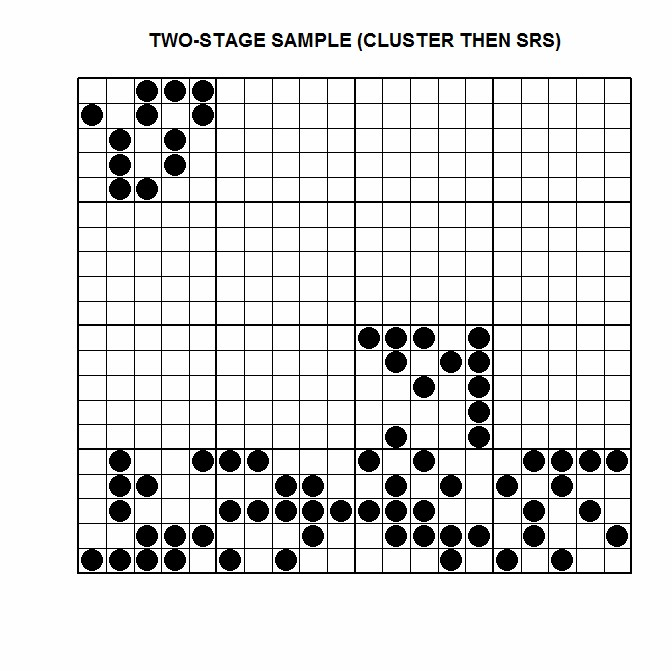The following diagram shows a two-stage sample.  In the first stage, 6 of the 16 available clusters were randomly sampled.  In the second stage, 12 units were systematically sampled from each cluster, taking every second unit.